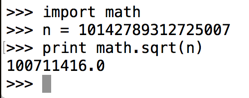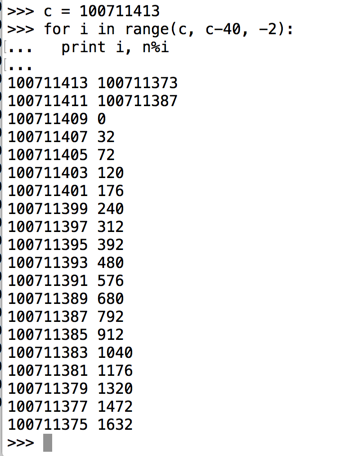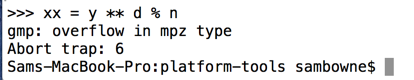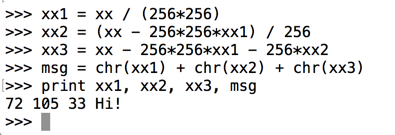# Proj RSA2: Cracking a Short RSA Key (15 pts.)

What you need:
• A Mac or Linux computer with Python.

## Purpose

To break into RSA encryption without prior knowledge of the private key. This is only possible for small RSA keys, which is why RSA keys should be long for security.

## Summary

Here's a diagram from the textbook showing the RSA calculations.## Problem Statement

Meghan's public key is (10142789312725007, 5). Find her private key.

## 1. Factoring n

### Finding the Square Root of n

n = 10142789312725007. This is the product of two prime numbers, p and q.

How large are p and q? Well, they can't both be larger than the square root of n, or they'd be larger than n when multiplied together.

Start Python in interactive mode. On a Mac or Linux box, you can do that by typing this command into a Terminal window:

``` python ```
Execute these commands:
```import math n = 10142789312725007 print math.sqrt(n) ```
The square root prints out, as shown below.### Displaying More Decimal Places

It's not clear from that output whether the result is an integer, or just rounded off to one decimal place. To see more decimal places, we'll use the repr() function.

Execute this command:

```print repr(math.sqrt(n)) ```
Now more decimal places appear, as shown below.### Testing 20 Candidates

So one of the prime factors must be a prime number less than 100711415. All we have to do is try dividing n by odd numbers starting at 100711413 and going down until we get an integer result. (We don't need to test 100711415 because it's divisible by 5 and therefore not a prime number.)

A good way to do this is to calculate n mod c, where c is a candidate. If c is a factor of n, the result will be zero.

We can test the first 20 candidates with a for loop.

Execute these commands:

```c = 100711413 for i in range(c, c-40, -2): print i, n%i ```
Press Enter twice after the last command to terminate the loop.

The third candidate is the winner, with a remainder of zero, as shown below.### Calculating q

We now know p and we can calculate q.

Execute these commands:

```p = 100711409 q = n / p print p, q, n, p*q, n - p*q ```
The calculation worked, so the last value is zero, as shown below.## 2. Compute phin = (p-1) * (q-1)

Execute these commands:
```phin = (p-1) * (q-1) print p, q, n, phin ```
The parameters print out, as shown below.## 3. Compute Private Key d

We need to find a d with this property:
(d * e) mod phin = 1
We know that e = 5 from the Problem Statement.

It's not obvious how to find d, but there's a simple way to do it in Python, using the "gmpy> library.

Open a new Terminal window, not the one running Python, and execute this command to download and install a few dependencies and gmpy:

```brew install gmp mpfr mpc pip install gmpy ```

In the Terminal window running python, execute these commands.

```e = 5 import gmpy d = gmpy.invert(e, phin) print d, e, d*e %phin ```
We get the value of d, and, to verify it, we see that d*e %phin is indeed 1, as shown below.## 4. Encrypting a Message

### Encoding the Message as a Number

Cueball wants to send Meghan this message:
```Hi! ```
We can only send numbers. Let's convert that message to three bytes of ASCII and then interpret it as a 24-bit binary value.

In the Terminal window running python, execute these commands.

```x1 = ord('H') x2 = ord('i') x3 = ord('!') x = x1*256*256 + x2*256 + x3 print x ```
We get the value of x, as shown below.### Encrypting the Message with the Public Key

A public key contains two numbers: n and e. To encrypt a message x, use this formula:Execute these commands:

```y = x ** e % n print y ```
The encrypted message appears, as shown below.## 5. Decrypting a Message

To decrypt a message, use this formula:Execute these commands:
```xx = y ** d % n print xx ```
Python crashes, as shown below. It cannot handle such large numbers.To compute such a number, we must use the pow() function.

Execute these commands to restart python and restore all the values we calculated previously:

```python n = 10142789312725007 p = 100711409 q = 100711423 phin = (p-1) * (q-1) e = 5 import gmpy d = gmpy.invert(e, phin) x1 = ord('H') x2 = ord('i') x3 = ord('!') x = x1*256*256 + x2*256 + x3 y = x ** e % n ```
Your screen should look like the image below.Let's try that decryption again with the pow() function. Execute these commands:
```xx = pow(y, d, n) print xx ```
Now it works, showing our original message in numerical form.### Converting the Message to Readable Text

To convert that number back to letters, execute these commands:
```xx1 = xx / (256*256) xx2 = (xx - 256*256*xx1) / 256 xx3 = xx - 256*256*xx1 - 256*xx2 msg = chr(xx1) + chr(xx2) + chr(xx3) print xx1, xx2, xx3, msg ```
Now it works, showing the original message in readable form, as shown below.## Challenge 2a: Encrypt "OBEY!"

Using the same system and keys, encrypt this message:
```OBEY!
```
Hint 1: The message, converted to a number, is 12 digits long and ends in 41.

Hint 2: The encrypted message is 16 digits long and ends in 81.

Use the form below to put your name on the WINNERS PAGE.

 Your Name (without spaces): Encrypted message:

## Challenge 2b: Message to Cueball

Cueball's public key is:
```(111036975342601848755221, 13)
```
Meghan sends this message to Cueball. Decrypt it.
```80564890594461648564443
```
Use the form below to put your name on the WINNERS PAGE.
 Your Name (without spaces): Cleartext Message:

## Challenge 3: Message to Rob

Rob public key is:
```(1234592592962967901296297037045679133590224789902207663928489902170626521926687, 5557)
```
Meghan sends this message to Rob. Decrypt it.
```272495530567010327943798078794037733865151017104532777645776936985235709526002
```
Hint: make square root calculations more precise.

Use the form below to put your name on the WINNERS PAGE.

 Your Name (without spaces): Cleartext Message:

## Sources

Cracking short RSA keys
Posted 3-31-16 by Sam Bowne